Ex 13.1

Chapter 13 Class 12 Probability
Serial order wise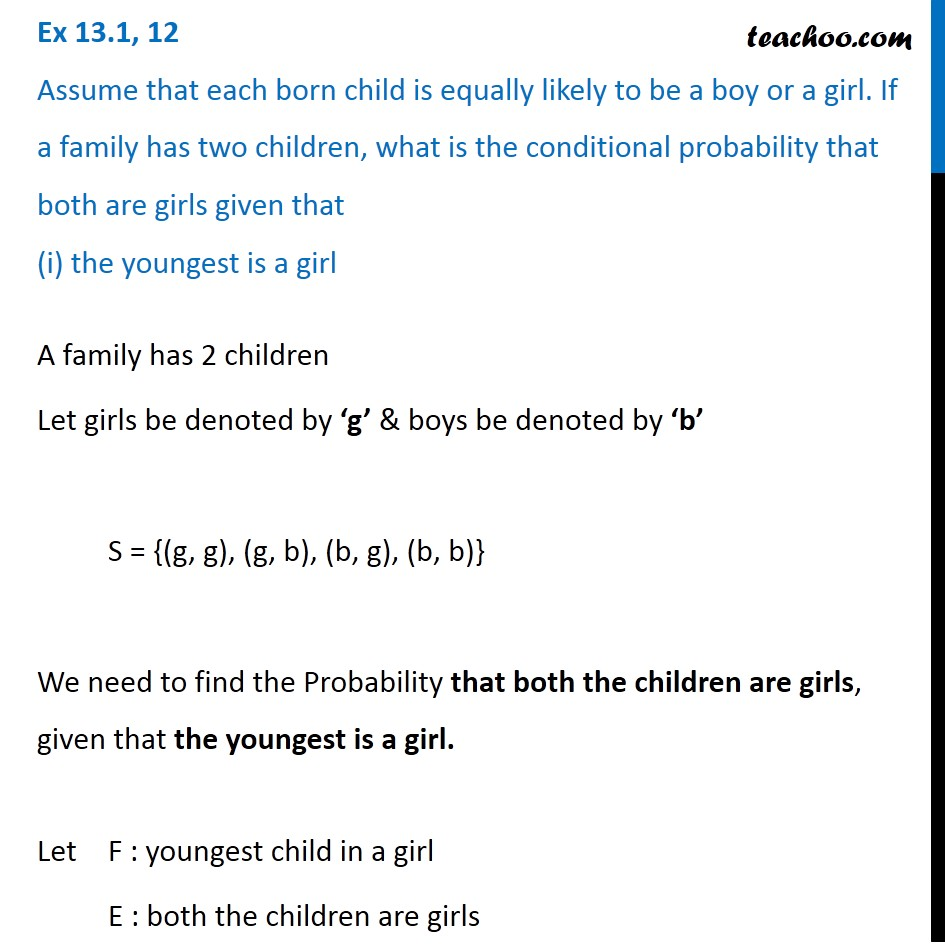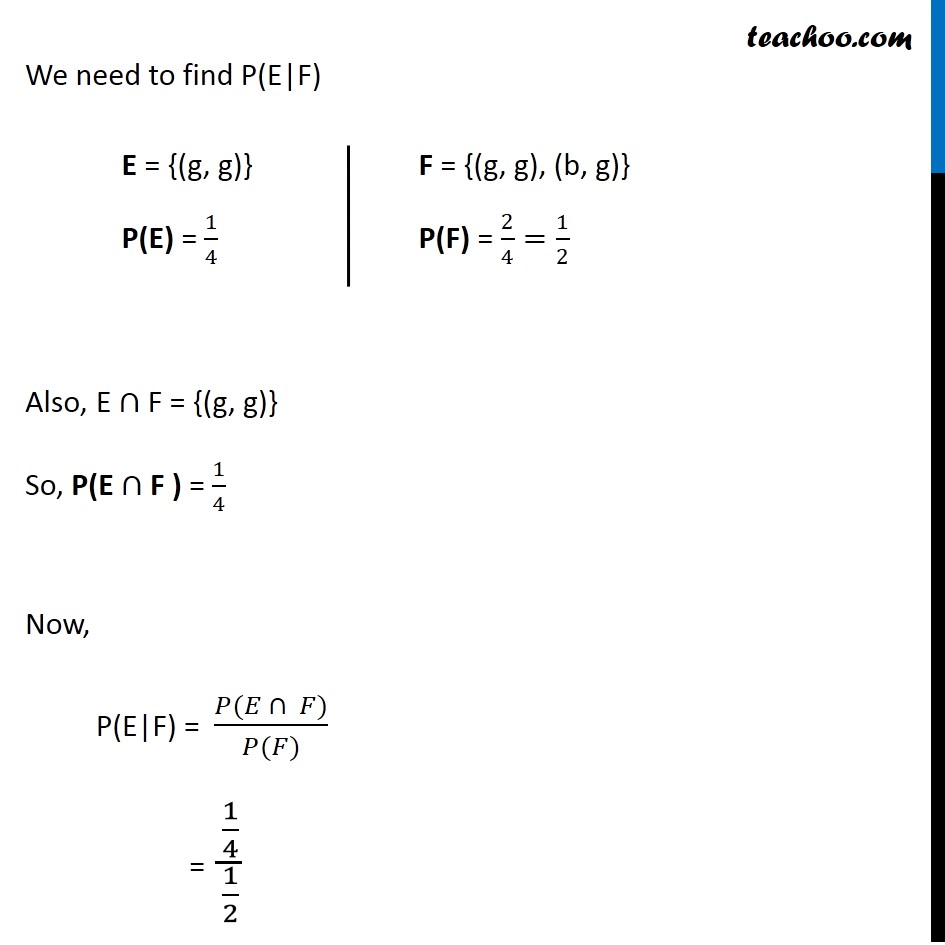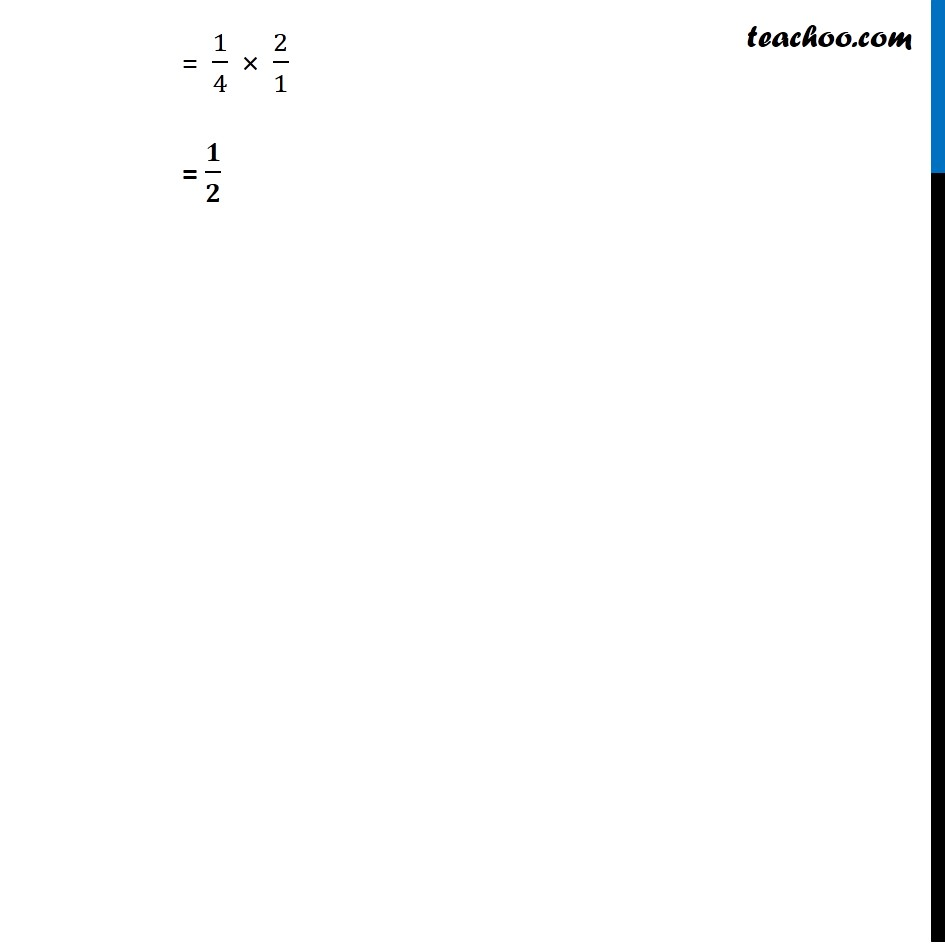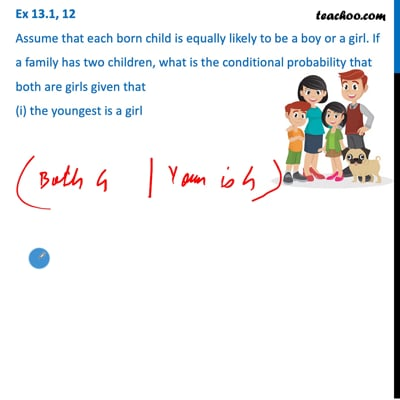This video is only available for Teachoo black users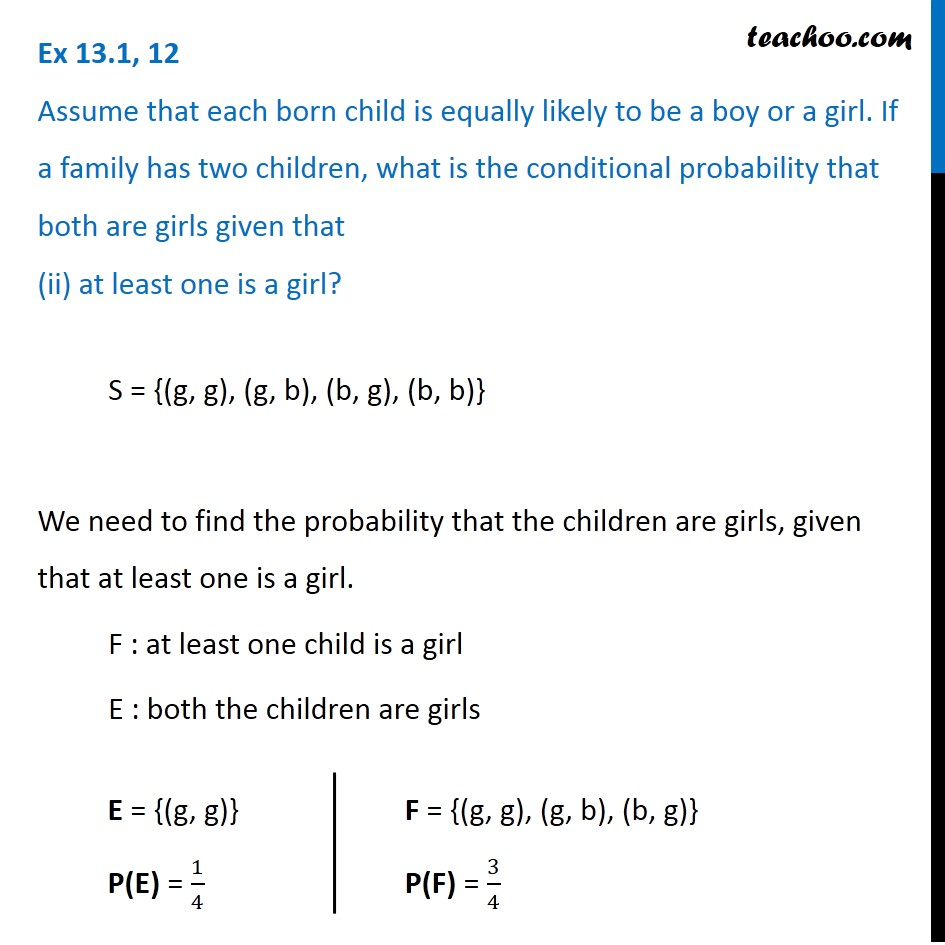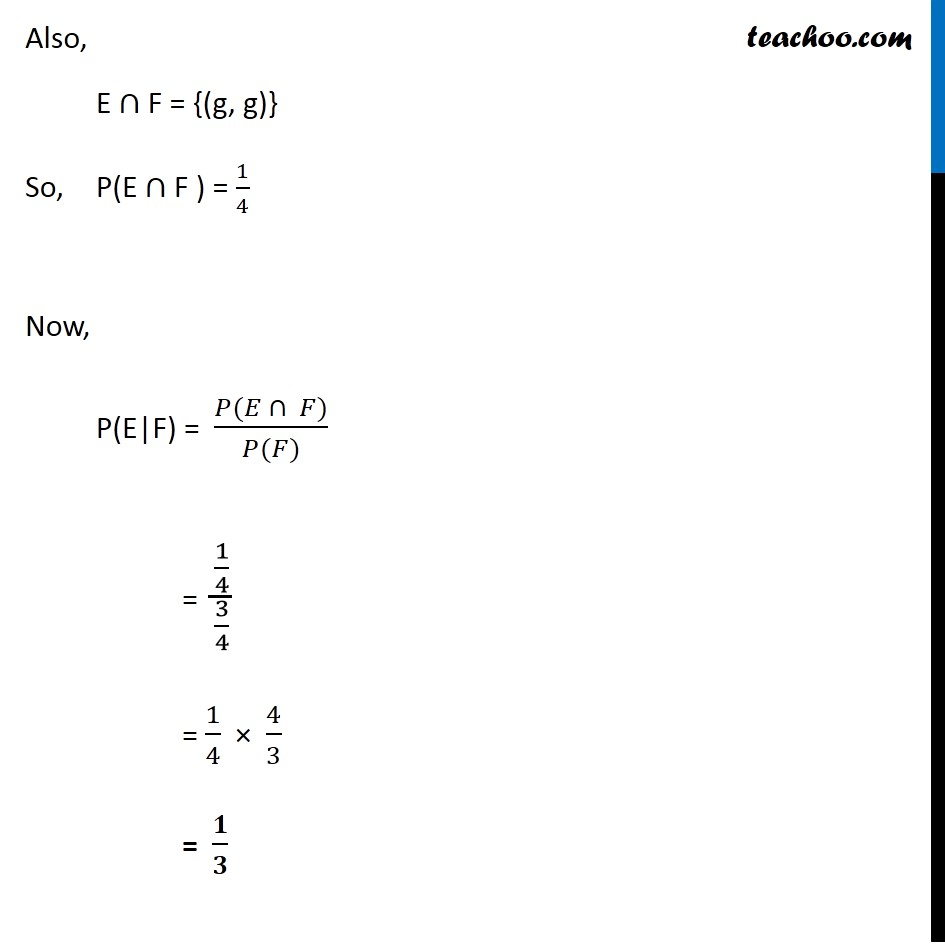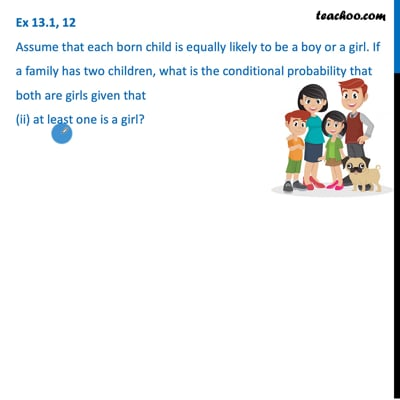This video is only available for Teachoo black users

Solve all your doubts with Teachoo Black (new monthly pack available now!)

### Transcript

Ex 13.1, 12 Assume that each born child is equally likely to be a boy or a girl. If a family has two children, what is the conditional probability that both are girls given that (i) the youngest is a girlA family has 2 children Let girls be denoted by ‘g’ & boys be denoted by ‘b’ S = {(g, g), (g, b), (b, g), (b, b)} We need to find the Probability that both the children are girls, given that the youngest is a girl. Let F : youngest child in a girl E : both the children are girls We need to find P(E|F) Also, E ∩ F = {(g, g)} So, P(E ∩ F ) = 1/4 Now, P(E|F) = (𝑃(𝐸 ∩ 𝐹))/(𝑃(𝐹)) = (1/4)/(1/2) E = {(g, g)} P(E) = 1/4 F = {(g, g), (b, g)} P(F) = 2/4=1/2 = 1/4 × 2/1 = 𝟏/𝟐 Ex 13.1, 12 Assume that each born child is equally likely to be a boy or a girl. If a family has two children, what is the conditional probability that both are girls given that (ii) at least one is a girl? S = {(g, g), (g, b), (b, g), (b, b)} We need to find the probability that the children are girls, given that at least one is a girl. F : at least one child is a girl E : both the children are girls E = {(g, g)} P(E) = 1/4 F = {(g, g), (g, b), (b, g)} P(F) = 3/4 Also, E ∩ F = {(g, g)} So, P(E ∩ F ) = 1/4 Now, P(E|F) = (𝑃(𝐸 ∩ 𝐹))/(𝑃(𝐹)) = (1/4)/(3/4) = 1/4 × 4/3 = 𝟏/𝟑